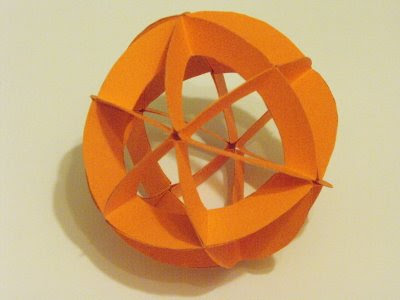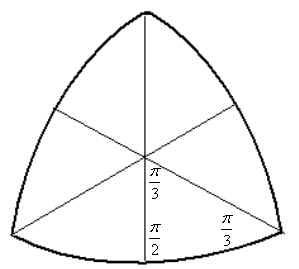## Wednesday, April 22, 2009

### Inflated Numbers

The word 'trillion' comes up with increasing regularity, and it seems that we are going to have to get used to finding the right words for larger and larger numbers.

In 1827, the textbook "Adam's New Arithmetic" (a pdf of the 1810 edition is available here) discussed numeration as far as the quadrillions - a real term, unlike gazillion.Thankfully, wikipedia takes us all the way to centillion.

The name for 10^x makes more sense if you consider n = x/3 - 1. So, for "octodecillion", which is 10^57, we look at 57/3 -1 = 18, which is octo (8) + deci (10). The "divide by three" occurs because each name gets three positions (e.g. millions, tens of millions, hundreds of millions). The "minus one" occurs since the naming scheme begins with millions, rather than thousands.

## Wednesday, April 15, 2009

### Quasi-regular Rhombic Tiling and PolyhedronIt could be argued that the square is the 'nicest' rhombus, but the rhombus with angles of 60 and 120 degrees seems nicer still. One of the nice things about the 60/120 rhomb are the plane tilings that can be constructed from it. One of these tilings is the 'tumbling blocks' tiling shown at the top of the post, in which at some points you see 'cubes' (around the degree 3 vertices), while at others you see 'flowers' (around the degree 6 vertices). Because of the two different types of vertices, this is known as a quasi-regular rhombic tiling. (Another tiling that uses the 60/120 rhomb is this one.)

If you want to build a polyhedron that resembles the tumbling block tiling, one method is to reduce the number of petals in your flowers to 5, and then stretch your 60/120 rhombs until they are have angles of 63.435 and 116.565 degrees. Thirty of these rhombs arranged around vertices of degree 3 and 5 produces a quasi-regular polygon known as the rhombic triacontahedron.

The image above is of a model that was built using rhombic units printed onto card stock. (A printable file is here).

Despite the apparent ugliness of the 63.435 and 116.565 degree angle-measurements, our nice 60/120 rhomb has been, arguably, stretched into an even nicer one - a 'golden rhombus,' so called because the ratio of the diagonals is equal to the golden ratio.

The dual of the rhombic triacontahedron is the archemedian polyhedron known as the icosidodecahedron. The image below is of a somewhat decorated model that was built using the units described here.

## Monday, April 13, 2009

### Species & Culture

"... the very greatest mathematical achievements have been due, not alone to the peering, microscopic, histologic vision of men like Weierstrass, illuminating the hidden recesses, the minute and intimate structure of logical reality, but to the larger vision also of men like Klein who survey the kingdoms of geometry and analysis for the endless variety of things that flourish there..."
from Mathematics, by C. J. Keyser,
Columbia University Press, 1907. p32

The quote above, from a lecture given in 1907, refers to a division of mathematical labor that is still being discussed today. Freeman Dyson's Birds and Frogs and Timothy Gowers's The Two Cultures of Mathematics are two recent articles elaborate on the rift that Keyser pointed out: that there are two distinctly different approaches to doing mathematics - an approach from above, favored by the bird-like theoreticians, and an approach from below, taken by frog-like problem solvers.

For non-mathematicians, the Gowers and Dyson articles provide windows into the usually opaque world of how mathematicians work and how they think about what they do. That mathematicians have differing views on what doing mathematics means would surprise many; that some view their work in almost heroic terms (Dyson's 'birds' and Gowers's 'theory builders') would surprise even more. The orientation that Dyson describes as 'frog' and that Gowers describes as 'problem solver' (categories into which they fit themselves, respectively) would probably be close to what the general public sees as the mathematical mind-set, although many would still be surprised that these problem solving frogs are attacking genuinely new problems.

Often when discussing how mathematicians view their work, we attempt to sort them by their 'philosophy of mathematics' - do they see themselves as neo-platonists, as formalists, as constuctivists? And, related to this, do they see themselves as discovering or inventing? Both the Dyson and Gowers articles avoid this over-used characterization of mathematicians via philosophy. Much more productively, both authors choose to focus on how mathematicians actually work, and on what they actually do. Focusing on actual mathematical practice and on the culture that grows up around it, as these articles do, provides much more insight into how mathematicians view their work and understand each other.

Through their articles, Dyson and Gowers are striving for a reconciliation between the two camps of mathematicians that they describe. The idea that mathematicians may fail to value the contributions of collegues who approach their work differently may come as another surprise for many non-mathematicians - the unfortunate reality is that, as in all fields, there are plenty of hierarchies and cliques in the world of mathematics. Gowers notes that areas of mathematics that are associated more with 'problem solving' (like combinatorics) receive less prestige than those that are perceived to be theoretically richer, and that these perceptions are often based on misconception. (The disdain goes both ways, of course - category theory is still labeled as 'abstract nonsense,' a label only sometimes jokingly applied.) Both authors end on notes that call out for a greater awareness and sympathy for how the two spiecies, or cultures, of mathematicians can work together so that mathematics can advance collectively.

## Wednesday, April 8, 2009

### Spherical TetrahedronImagine a tetrahedron centered inside a sphere. If you were to project the edges of the tetrahedron out from the center so that they touched the surface of the sphere, the edges would cut the sphere in arcs that lie on the sphere's 'great circles' (the largest possible circles drawn on the surface of the sphere - circles whose radii are the same as the radius of the sphere).

The image at the top of this post shows a model of a sphere with the six great circles formed by the projected edges of a tetrahedron sitting at its center.

The model, described on pages 4 and 5 of Magnus Wennigers' Polyhedron Models, is easy to construct using the template shown below. Printing copies of the template onto card-stock and gluing them together works well. This pdf file has 10 arcs on a single page for printing (you will need 24 arcs in total).Each arc is folded and glued to form a spherical triangle, 24 of the spherical triangles glued together will form the sphere.

The diagram below (based on Fig.2, p.5, in Wenniger) shows how to assemble 6 of the triangles into one 'face' of the spherical tetrahedron. Four of the faces can be assembled into the 'spherical tetrahedron.' When assembling, you should make sure that you align the triangles so that they form great circles.Spherical triangles, as you can tell from the diagram, do not follow the rule of having their interior angles sum to pi (180 degrees), as plane triangles do. See wikipedia and mathworld for more on the relations that govern spherical triangles.

The arc is divided into angles of 54deg&44min, 70deg&32min, 54deg&44min, as described by Wenniger. The template was created in GSP.

## Thursday, April 2, 2009

### Hinged Triangle to Square TransformationThe construction above, which shows how an equilateral triangle can be cut, hinged, and transformed into a square is the very first entry in Hugo Stienhaus's Mathematical Snapshots. Constructing this hinged triangle/square in GSP turns out to be quite a nice activity.

Erik Demain's paper Hinged Dissection of Polyominoes and Polyforms takes this example as its starting point, develops the theory of hinged dissections, and shows how this example and others can be taken further.

The references in Demain's paper lead to a great site on geometric dissections (non-hinged), and to the work of Greg N. Frederickson (hinged, non-hinged, and folded dissections).

On Frederickson's site, among many great things, we find a link to a lesson plan that includes instructions on building a physical model of the triangle-square hinged dissection.